# Force - Where Does It Come From?

Okay, so you have some iron in a magnetic field. So what? Where does the force come from? When you hold two magnets and bring them together, you can feel the force but not see it. So it seems mysterious, but it must have something in common the more familiar forces, right?

This page briefly summarizes the physics of forces, along with some math that I can't explain. For a serious understanding you'll need to pursue a college physics course or textbook.

## Force Is the Gradient of Potential Energy

Force is the gradient of potential energy.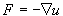This is true for anything: gravity, electric fields, mechanical force, springs and rubber bands, air pressure, magnetism. Anything you can think of!

The gradient operator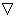tells how a function varies in different directions. It is a vector given by these two rules:

1. It points in the direction in which f(x, y, z) increases most rapidly;
2. Its magnitude is the rate of change of f(x, y, z) with respect to distance in that direction.

In short, the gradient tells you how to calculate directional derivatives.

If a system has a potential energy field, then it invariably generates forces. "Potential energy" is defined by the stored energy as a result of an object's position.

## Force In a Magnetic Field

The potential energy in a magnetic field iswhereis magnetic induction vector,
and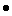is dot product,
and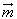is magnetic moment vector.

Recall that , i.e. magnetic induction vector B is equal to permeability µ multiplied by magnetic field vector H.

Therefore the energy density in a magnetic field isNote that force units depend on your choice of systems:

 MKS:CGS: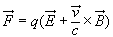Although the equations look similar, these are fundamentally different units. They are not basically comparable since they have different dimensions.

 < Previous Page 4 of 11 Next >